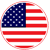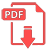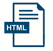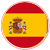# Home | Mathematics | Number Systems

## Number Rounding

Rounding a number means replacing it with a different number that is approximately equal to the original, but has a shorter, simpler, or more explicit representation; for example, replacing \$23.4476 with \$23.45, or the fraction 312/937 with 1/3, or the expression √2 with 1.414.

Rounding is often done to obtain a value that is easier to report and communicate than the original. Rounding can also be important to avoid misleadingly precise reporting of a computed number, measurement or estimate; for example, a quantity that was computed as 123,456 but is known to be accurate only to within a few hundred units is usually better stated as "about 123,500".

On the other hand, rounding of exact numbers will introduce some round-off error in the reported result. Rounding is almost unavoidable when reporting many computations – especially when dividing two numbers in integer or fixed-point arithmetic; when computing mathematical functions such as square roots, logarithms, and sines; or when using a floating-point representation with a fixed number of significant digits. In a sequence of calculations, these rounding errors generally accumulate, and in certain ill-conditioned cases they may make the result meaningless.EnglishSpanish